Learn all Concepts of Chapter 3 Class 10 (with VIDEOS). Check - Linear Equations in 2 Variables - Class 10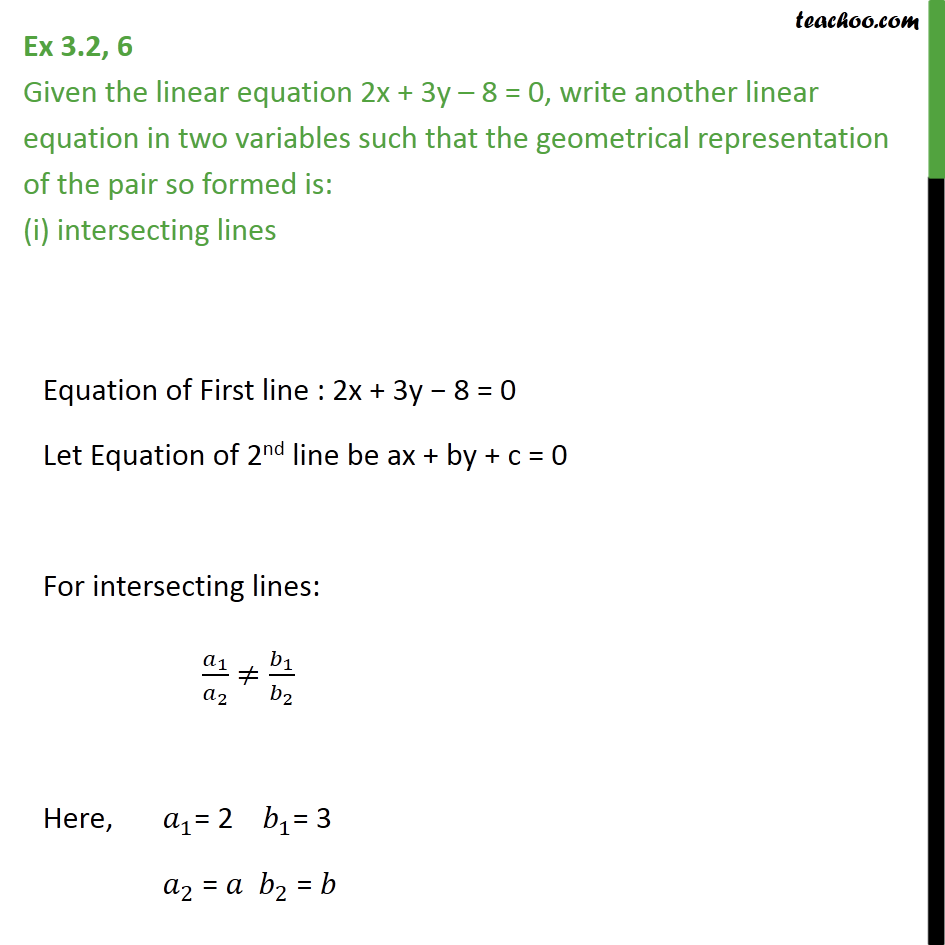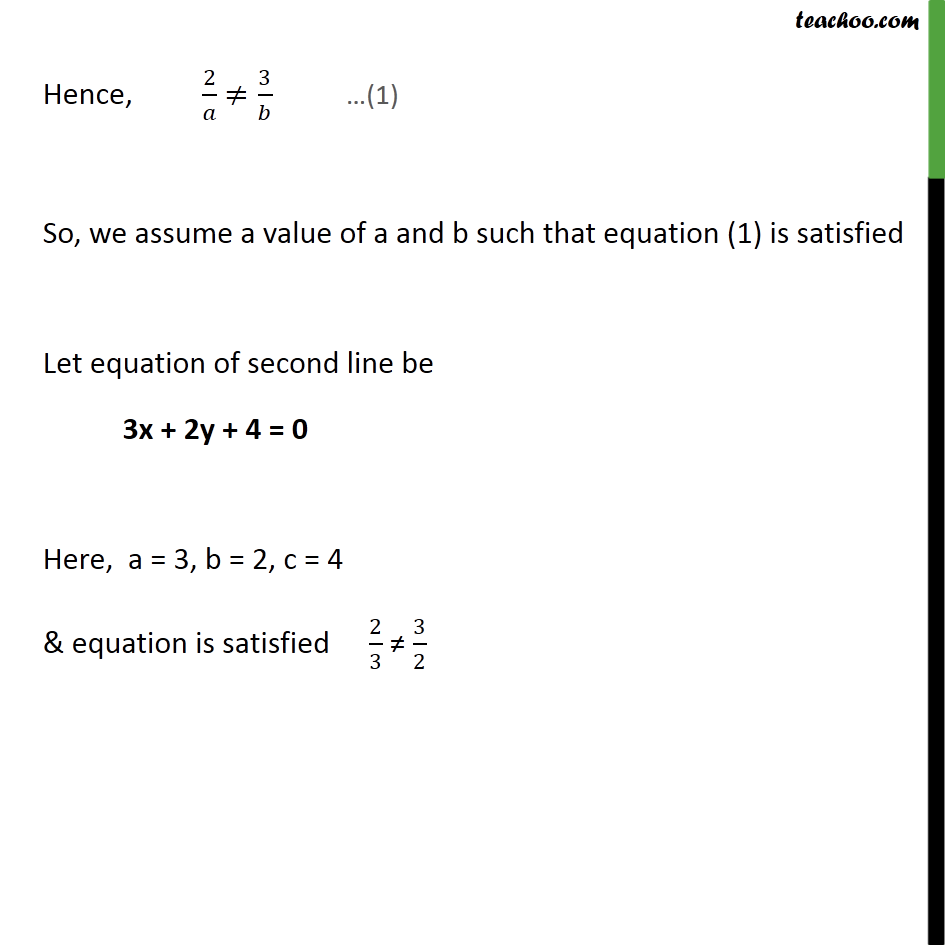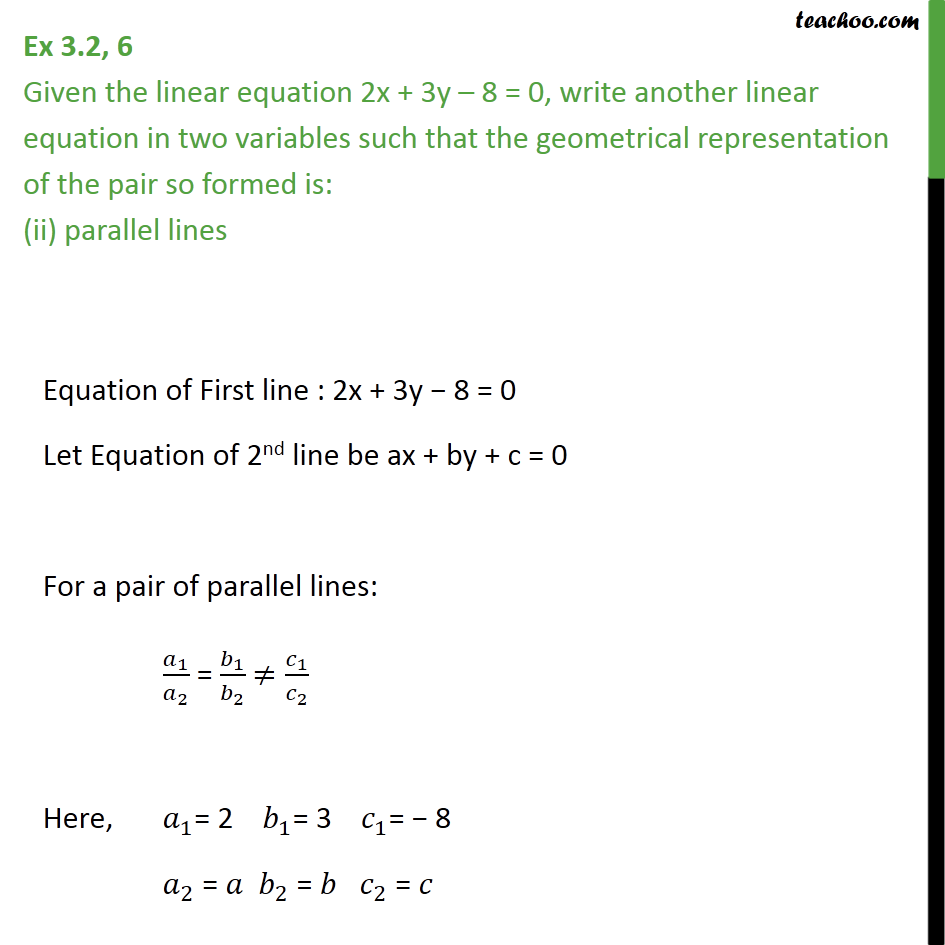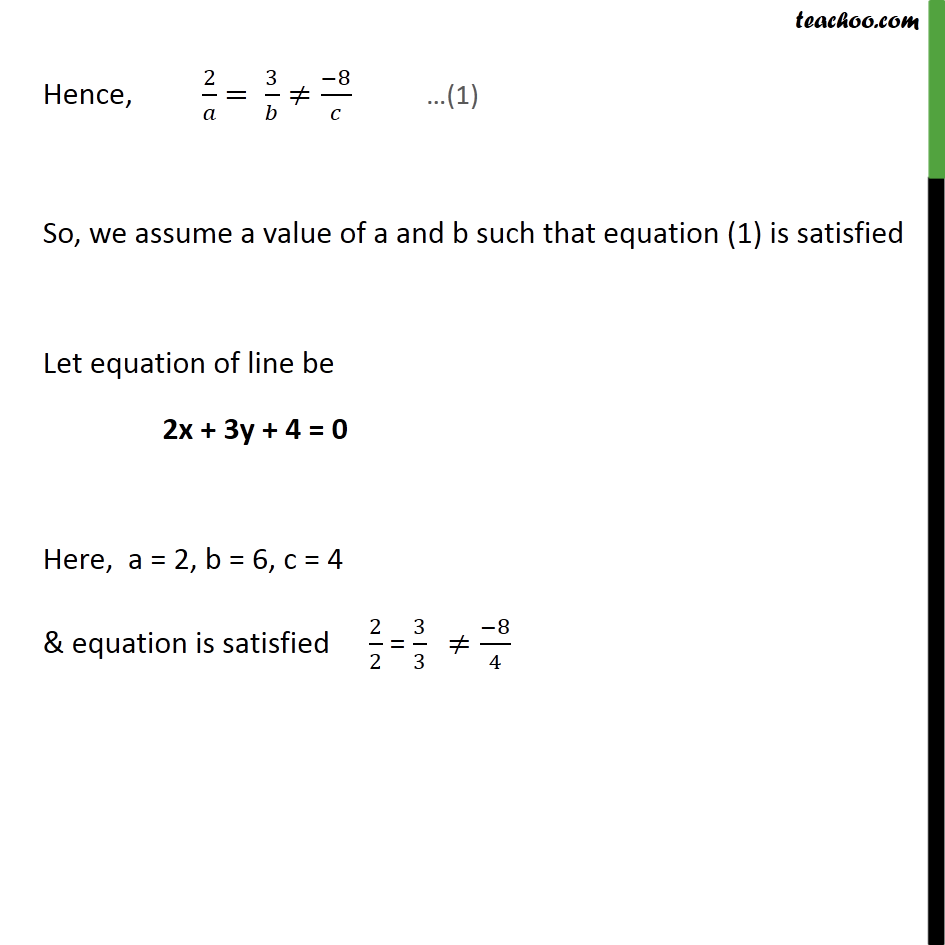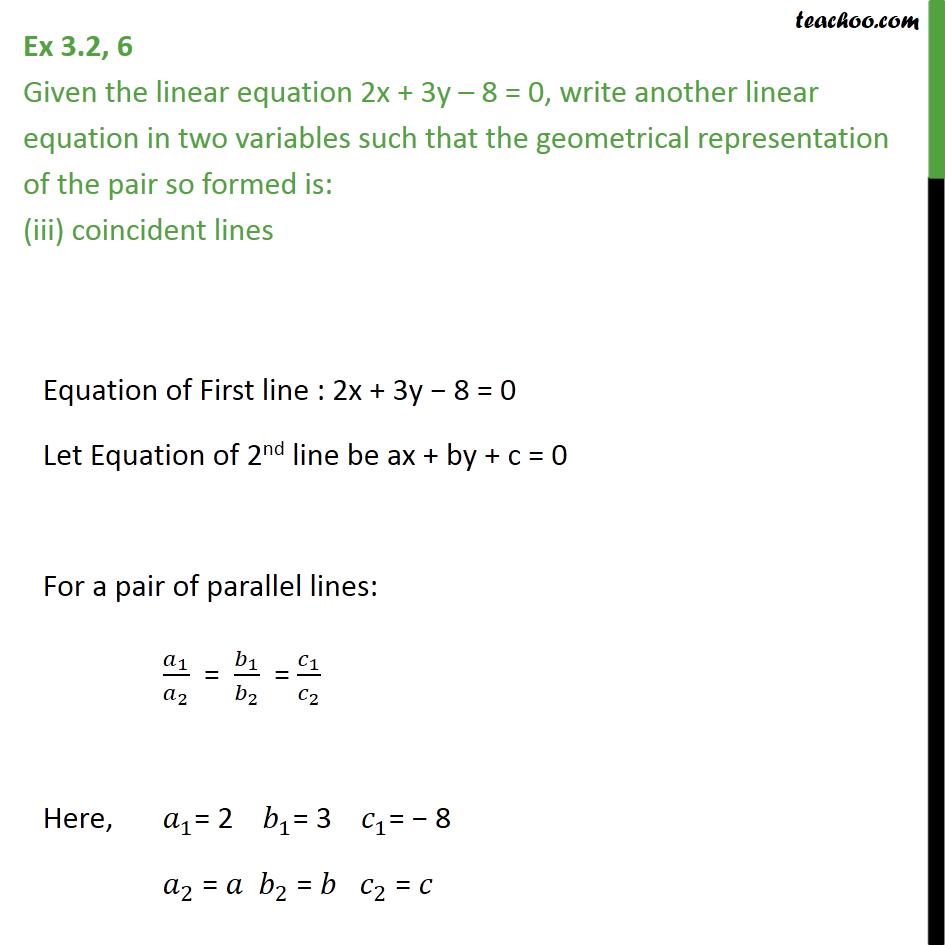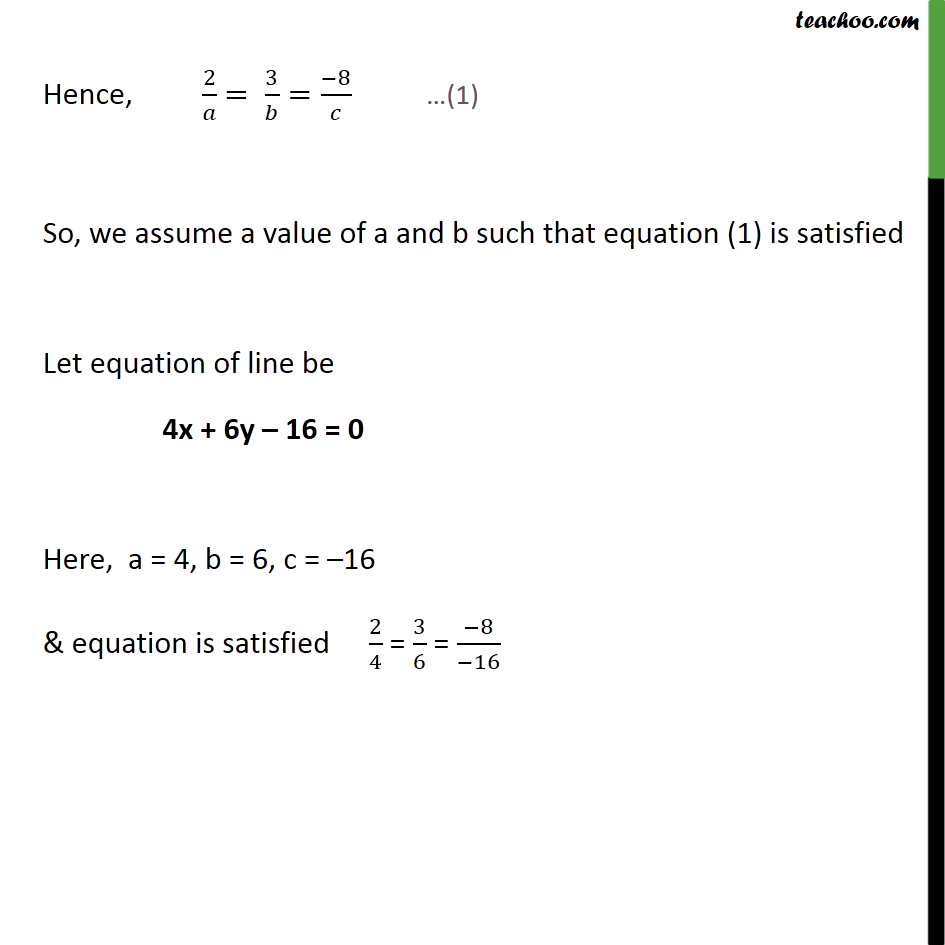1. Chapter 3 Class 10 Pair of Linear Equations in Two Variables
2. Serial order wise
3. Ex 3.2

Transcript

Ex 3.2, 6 Given the linear equation 2x + 3y – 8 = 0, write another linear equation in two variables such that the geometrical representation of the pair so formed is: (i) intersecting lines Equation of First line : 2x + 3y − 8 = 0 Let Equation of 2nd line be ax + by + c = 0 For intersecting lines: 𝑎_1/𝑎_2 ≠𝑏_1/𝑏_2 Here, 𝑎_1= 2 𝑏_1= 3 𝑎_2 = 𝑎 𝑏_2 = 𝑏 Hence, 2/𝑎 ≠3/𝑏 So, we assume a value of a and b such that equation (1) is satisfied Let equation of second line be 3x + 2y + 4 = 0 Here, a = 3, b = 2, c = 4 & equation is satisfied 2/3 ≠ 3/2 Ex 3.2, 6 Given the linear equation 2x + 3y – 8 = 0, write another linear equation in two variables such that the geometrical representation of the pair so formed is: (ii) parallel lines Equation of First line : 2x + 3y − 8 = 0 Let Equation of 2nd line be ax + by + c = 0 For a pair of parallel lines: 𝑎_1/𝑎_2 = 𝑏_1/𝑏_2 ≠𝑐_1/𝑐_2 Here, 𝑎_1= 2 𝑏_1= 3 𝑐_1= − 8 𝑎_2 = 𝑎 𝑏_2 = 𝑏 𝑐_2 = 𝑐 Hence, 2/𝑎 = 3/𝑏≠(−8)/𝑐 So, we assume a value of a and b such that equation (1) is satisfied Let equation of line be 2x + 3y + 4 = 0 Here, a = 2, b = 6, c = 4 & equation is satisfied 2/2 = 3/3 " "≠(−8)/4 Ex 3.2, 6 Given the linear equation 2x + 3y – 8 = 0, write another linear equation in two variables such that the geometrical representation of the pair so formed is: (iii) coincident lines Equation of First line : 2x + 3y − 8 = 0 Let Equation of 2nd line be ax + by + c = 0 For a pair of parallel lines: 𝑎_1/𝑎_2 = 𝑏_1/𝑏_2 = 𝑐_1/𝑐_2 Here, 𝑎_1= 2 𝑏_1= 3 𝑐_1= − 8 𝑎_2 = 𝑎 𝑏_2 = 𝑏 𝑐_2 = 𝑐 Hence, 2/𝑎 = 3/𝑏=(−8)/𝑐 So, we assume a value of a and b such that equation (1) is satisfied Let equation of line be 4x + 6y – 16 = 0 Here, a = 4, b = 6, c = –16 & equation is satisfied 2/4 = 3/6 = (−8)/(−16)

Ex 3.2